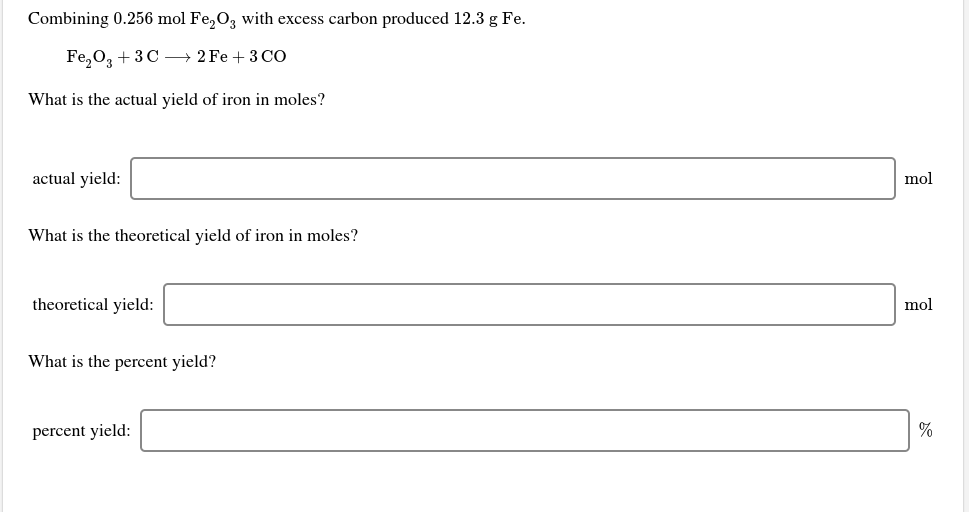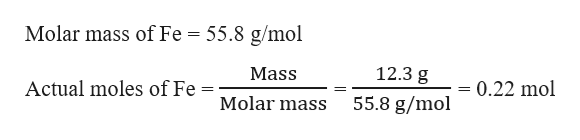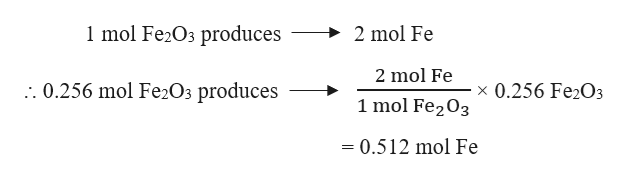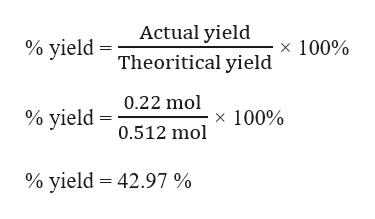# Combining 0.256 mol Fe,O, with excess carbon produced 12.3 g Fe.Fe,0, + 3C → 2 Fe + 3 COWhat is the actual yield of iron in moles?actual yield:molWhat is the theoretical yield of iron in moles?theoretical yield:molWhat is the percent yield?percent yield:

Question
1 views

i dont get any of thishelp_outlineImage TranscriptioncloseCombining 0.256 mol Fe,O, with excess carbon produced 12.3 g Fe. Fe,0, + 3C → 2 Fe + 3 CO What is the actual yield of iron in moles? actual yield: mol What is the theoretical yield of iron in moles? theoretical yield: mol What is the percent yield? percent yield: fullscreen
check_circle

Step 1

Given,

Moles of Fe2O3 = 0.256 mol

Mass of Fe produced = 12.3 g

The actual yield of iron in moles can be calculated as :help_outlineImage TranscriptioncloseMolar mass of Fe = 55.8 g/mol 12.3 g Mass Actual moles of Fe = 0.22 mol 55.8 g/mol Molar mass fullscreen
Step 2

The balanced chemical reaction is given as:

Fe2O3 + 3 C → 2 Fe + 3 CO

The theoretical yield of iron in moles can be calculated as :help_outlineImage Transcriptionclose1 mol Fe2O3 produces 2 mol Fe 2 mol Fe .. 0.256 mol Fe2O3 produces x 0.256 Fe2O3 1 mol Fe203 = 0.512 mol Fe fullscreen
Step 3

Therefore, the percent yield ...help_outlineImage TranscriptioncloseActual yield × 100% % yield Theoritical yield 0.22 mol % yield x 100% 0.512 mol % yield = 42.97 % fullscreen

### Want to see the full answer?

See Solution

#### Want to see this answer and more?

Solutions are written by subject experts who are available 24/7. Questions are typically answered within 1 hour.*

See Solution
*Response times may vary by subject and question.
Tagged in

### General Chemistry